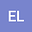loading page

On solutions of PDEs by using algebras
•## Abstract

The components of complex differentiable functions define solutions for the Laplace’s equation. In this paper we generalize this result; for each PDE of the form $Au_{xx}+Bu_{xy}+Cu_{yy}=0$ we give an affine planar vector field $\varphi$ and an associative and commutative 2D algebra with unit $\mathbb A$, with respect to which the components of all functions of the form $\mathcal L\circ\varphi$ define solutions for this PDE, where $\mathcal L$ is differentiable in the sense of Lorch with respect to $\mathbb A$. In the same way, for each PDE of the form $Au_{xx}+Bu_{xy}+Cu_{yy}+Du_x+Eu_y+Fu=0$, the components of the exponential function $e^{\varphi}$ defined with respect to $\mathbb A$, define solutions for this PDE. In the case of PDEs of the form $Au_{xx}+Bu_{xy}+Cu_{yy}+Fu=0$, sine, cosine, hyperbolic sine, and hyperbolic cosine functions can be used instead of the exponential function. Also, solutions for two dependent variables $3^{\text{th}}$ order PDEs and a $4^{\text{th}}$ order PDE are constructed.

#### Peer review status:UNDER REVIEW

26 Mar 2021Submitted to Mathematical Methods in the Applied Sciences
27 Mar 2021Assigned to Editor
27 Mar 2021Submission Checks Completed
03 Apr 2021Reviewer(s) Assigned